# MCAT Physical : Other Electrochemical Principles

## Example Questions

### Example Question #1 : Other Electrochemical Principles

A researcher is analyzing an electrolytic cell. In trial 1, he applies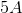of current for two hours to the cell and observes that the cathode produces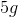of zinc metal. In trial 2, he applies the same amount of current but only for one hour. What will the researcher observe in the cathode during trial 2?

Same amount of zinc as trial 1 because the current stays the same

Smaller amount of zinc than trial 1 because the applied charge decreases

Same amount of zinc as trial 1 because the applied charge stays the same

Larger amount of zinc than trial 1 because the charge decreases

Smaller amount of zinc than trial 1 because the applied charge decreases

Explanation:

Faraday’s Law states that the amount of substance produced in a half-cell is dependent on the charge applied to the system; therefore, the more charge applied the more substance produced. The question states that the researcher applies the same amount of current, but for a shorter time, in trial 2. Recall the definition of current: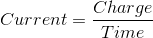This means that charge equals: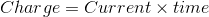Trial 1 and Trial 2 have the same current; however, trial 2 has a smaller time. This means that the charge applied in trial 2 is smaller than the charge applied in trial 1; therefore, according to Faraday’s law, the researcher must observe a smaller mass of zinc metal in trial 2.

### Example Question #22 : Electrochemistry

Faraday’s Law states that the amount of product in a cell __________ as the __________ increases.

increases . . . charge

increases . . . time

decreases . . . charge

decreases . . . time

increases . . . charge

Explanation:

Faraday’s Law states that the amount of product in a half cell is proportional to the charge applied to the cell; therefore, the amount of product in a cell increases as the charge increases. Faraday’s Law states nothing about the relationship between the amount of product and time.

### Example Question #23 : Electrochemistry

Which of the following substances cannot conduct electricity in water?

I. Sodium chloride

II. Iron (II) carbonate

III. Glucose

II and III

I and II

I only

II only

II and III

Explanation:

The question asks you to pick substances that cannot conduct electricity. Recall that molecules that conduct electricity in solution are called electrolytes. In a chemical solution, a substance will conduct electricity if it can dissociate into ions; therefore, you are looking for molecules that will not dissociate into ions in water.

Sodium chloride, or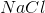, is an ionic compound that will dissociate into sodium ions and chlorine ions; therefore, sodium chloride will conduct electricity in water.

Iron (II) carbonate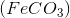is an insoluble compound in water. This means that it will not dissociate into ions and will not conduct electricity. Remember that most carbonates are insoluble in water.

Glucose is soluble in water because of its polar hydroxyl groups; however, it does not dissociate into ions. This means that glucose does not conduct electricity.

### Example Question #4 : Other Electrochemical Principles

Listed below are three substances that dissolve in water.

I. Acetic acid

II. Acetone

III. Hydrochloric acid

Which of the following correctly lists the substances in decreasing order of electrical conductivity?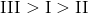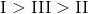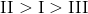Explanation:

Electrical conductivity is a measure of the ability of a substance to conduct electricity. A substance with a high electrical conductivity will easily conduct electricity, and a substance with low electrical conductivity will not conduct electricity.

In solution, a substance can conduct electricity if it can dissociate into ions. Of the three substances listed, hydrochloric acid is likely to have the highest electrical conductivity. Recall that hydrochloric acid (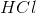) is a strong acid; therefore, it will completely dissociate into hydrogen and chlorine ions in water.

The substance with the second highest electrical conductivity is acetic acid. Although acetic acid is an acid, it is a weak acid. This means that the acetic acid will not completely dissociate into ions in water. If you have the same concentration of hydrochloric acid and acetic acid, hydrochloric acid will produce more ions in water and will be able to conduct more electricity; therefore, hydrochloric acid has a higher electrical conductivity than acetic acid.

Acetone is soluble in water; however, it does not dissociate into ions and will have the lowest electrical conductivity.

The correct order of electrical conductivity is: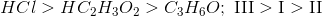.